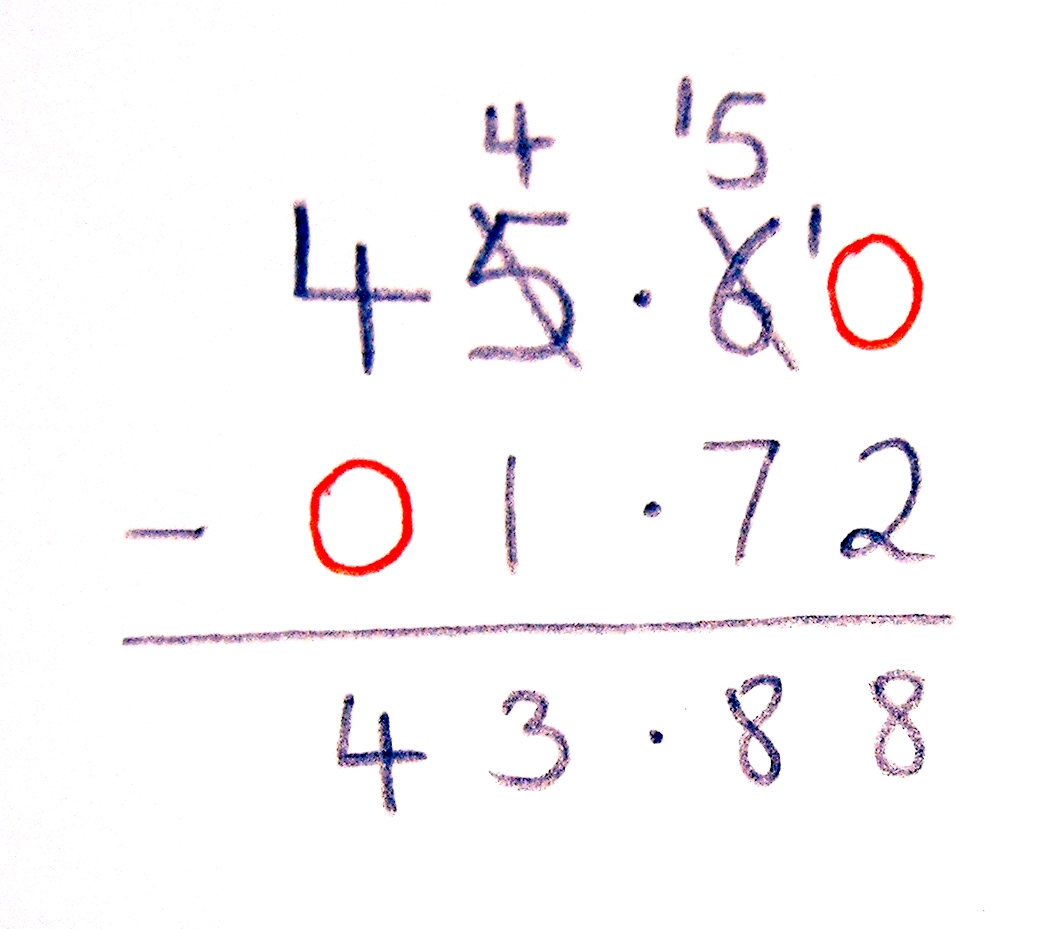The Singing Hedgehog Guide to:
Decimal Subtraction 1

When we subtract we still must have numbers
from the same column above each other so we:

LINE UP THE DECIMAL POINTS!

Now we MUST put in extra zeroes. Here is an example:

45.6 - 1.72

This becomes 45.20 -
01.62:We can see that the extra zero in the top
number gives us somewhere to 'borrow' to.

This is why we MUST put the extra zero
on the first number.
(The other zero is helpful but not essential)

Now you need to practise: